https://doi.org/10.5194/mr-2-251-2021
Special issue:
https://doi.org/10.5194/mr-2-251-2021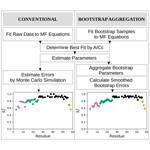# Bootstrap aggregation for model selection in the model-free formalism

Timothy Crawley and Arthur G. Palmer III
Abstract

The ability to make robust inferences about the dynamics of biological macromolecules using NMR spectroscopy depends heavily on the application of appropriate theoretical models for nuclear spin relaxation. Data analysis for NMR laboratory-frame relaxation experiments typically involves selecting one of several model-free spectral density functions using a bias-corrected fitness test. Here, advances in statistical model selection theory, termed bootstrap aggregation or bagging, are applied to 15N spin relaxation data, developing a multimodel inference solution to the model-free selection problem. The approach is illustrated using data sets recorded at four static magnetic fields for the bZip domain of the S. cerevisiae transcription factor GCN4.

Share
Dates
1 Introduction

Since the original publications in the early 1980s, the model-free formalism of and the related two-step approach of have served as starting points for extracting dynamical information about macromolecules from NMR spin relaxation data. The original approaches represented intramolecular dynamics using a single generalized order parameter and effective correlation time. In the ensuing decades, increasingly complex models have offered a more refined understanding of internal and overall molecular motions. Extended model-free formalisms characterize intramolecular dynamics using generalized order parameters and effective correlation times for more than one timescale (usually two) . Related approaches employ discrete or continuous distributions to more fully capture the range of intramolecular correlation times . Other strategies employ physical models or atomistic molecular dynamics simulations for overall rotational diffusion and internal conformational fluctuations, to more directly link the NMR phenomena to underlying physical processes . The availability of extended model-free formalisms, or other approaches with variable numbers of parameters, has created a further dilemma: should a data analysis protocol extract the most exacting information justified by the data or employ the model most robust to experimental variation?

Several authors have addressed model selection by employing the principle of parsimony or Occam's razor . These approaches seek to identify the simplest model that explains the data within experimental uncertainties by applying various bias-correcting penalties to the fitness statistic, e.g., F statistic, Akaike information criterion (AIC), or Bayesian information criterion (BIC). These corrections alone often fall short of producing robust inferences and may yield parameter values susceptible to instability in both simulated and real-world replicates. In these situations, the model selection process has failed the principle of “worrying selectively”. This criterion suggests, “Since all models are wrong, the scientist must be alert to what is importantly wrong.” (Box1976).

To illustrate the issue more concretely, a typical data analysis protocol uses a nonlinear weighted least-squares algorithm to fit experimental spin relaxation data with a set of model-free spectral density functions . The resulting χ2 residual sum-of-squares variables are penalized for the number of adjustable parameters in each model function, the model with the lowest penalized residual sum-of-squares is selected as optimal, and the best-fit parameters of the model are reported. However, this procedure is subject to model selection error: random statistical variation in the experimental data may lead to one model chosen as optimal for a given data set, but another model, with different set of parameters, may be selected if the experimental data were replicated, with consequent different random variation. The problem of joint model selection and parameter estimation has been explored elegantly by and by .

The present paper addresses model selection error by using the approach of bootstrap aggregation or bagging. This concept originated from a desire to improve the performance of machine learning algorithms. Thus, Breiman showed that predictor accuracy and stability improved when averaging predictor values obtained from bootstrap replicates of the original training set (Breiman1996). Buja and Stuetzle subsequently extended the use of bagging to generalized statistical analysis and showed sampling with and without replacement yield equivalent improvements . The approach and notation of Efron are used in the following (Efron2014).

Bootstrap aggregation improves parameter stability; consequently, the resulting variations in model-free parameter values, for example between atomic sites or functional states in a given macromolecule, are more likely to be biologically or chemically meaningful. Although applicable to most model selection situations, bootstrap aggregation exhibits the most pronounced benefits when the data justify two distinct models with similar degrees of certainty.

Bootstrap aggregation for model-free analysis of NMR spin relaxation rate constants is illustrated by application to backbone amide 15N spin relaxation data that have been recorded at 1H magnetic fields of 600, 700, 800, and 900 MHz for the bZip domain of the S. cerevisiae transcription factor GCN4 by Gill and coworkers .

2 Theory

In the following, the notation used by Efron is rephrased in terms appropriate for NMR spin relaxation data (Efron2014). Laboratory-frame nuclear spin relaxation rate constants for backbone 15N spins can be transformed into sets of spectral density function values, J(ω), in which ω is an eigenfrequency of the spin system . Laboratory-frame 15N relaxation rate constants, typically R1, R2, and the steady-state nuclear Overhauser enhancement (NOE), recorded at a single static magnetic field yield estimates of J(0), J(ωN), and J(0.87ωH), in which ωN and ωH are the 15N and 1H Larmor frequencies. Thus, the number of spectral density values N=3G, in which G is the number of static magnetic fields utilized. In the present application, G=4. The set of experimental spectral densities is described using the following notation:

$\begin{array}{}\text{(1)}& \mathbit{y}=\mathit{\left\{}{y}_{j}\mathit{\right\}}=\mathit{\left\{}{y}_{\mathrm{1}},{y}_{\mathrm{2}},\mathrm{\dots },{y}_{N}\mathit{\right\}}\phantom{\rule{0.125em}{0ex}},\end{array}$

in which the yj=J(ωj) are ordered in increasing values of ω. The values of J(0) are ordered additionally by increasing values of the static magnetic field. The experimental data sets utilized in the present work are not affected by chemical exchange contributions to spin relaxation, but such contributions can be taken into account from the field dependence of transverse relaxation rate constants prior to the model-free analysis .

The extended model-free spectral density function used to fit 15N spin relaxation data is given by the following:

$\begin{array}{}\text{(2)}& \begin{array}{rl}J\left(\mathit{\omega }\right)=\frac{\mathrm{2}}{\mathrm{5}}\left[& \frac{{S}_{\mathrm{f}}^{\mathrm{2}}{S}_{\mathrm{s}}^{\mathrm{2}}{\mathit{\tau }}_{m}}{\left(\mathrm{1}+{\mathit{\omega }}^{\mathrm{2}}{\mathit{\tau }}_{m}^{\mathrm{2}}\right)}+\frac{{S}_{\mathrm{f}}^{\mathrm{2}}\left(\mathrm{1}-{S}_{\mathrm{s}}^{\mathrm{2}}\right){\mathit{\tau }}_{\mathrm{1}}}{\left(\mathrm{1}+{\mathit{\omega }}^{\mathrm{2}}{\mathit{\tau }}_{\mathrm{1}}^{\mathrm{2}}\right)}\\ & \phantom{\rule{0.25em}{0ex}}\phantom{\rule{0.25em}{0ex}}+\frac{\left(\mathrm{1}-{S}_{\mathrm{f}}^{\mathrm{2}}\right){S}_{\mathrm{s}}^{\mathrm{2}}{\mathit{\tau }}_{\mathrm{2}}}{\left(\mathrm{1}+{\mathit{\omega }}^{\mathrm{2}}{\mathit{\tau }}_{\mathrm{2}}^{\mathrm{2}}\right)}+\frac{\left(\mathrm{1}-{S}_{\mathrm{f}}^{\mathrm{2}}\right)\left(\mathrm{1}-{S}_{\mathrm{s}}^{\mathrm{2}}\right){\mathit{\tau }}_{\mathrm{3}}}{\left(\mathrm{1}+{\mathit{\omega }}^{\mathrm{2}}{\mathit{\tau }}_{\mathrm{3}}^{\mathrm{2}}\right)}\right],\end{array}\end{array}$

in which ${\mathit{\tau }}_{\mathrm{1}}^{-\mathrm{1}}={\mathit{\tau }}_{m}^{-\mathrm{1}}+{\mathit{\tau }}_{\mathrm{s}}^{-\mathrm{1}}$, ${\mathit{\tau }}_{\mathrm{2}}^{-\mathrm{1}}={\mathit{\tau }}_{m}^{-\mathrm{1}}+{\mathit{\tau }}_{\mathrm{f}}^{-\mathrm{1}}$, ${\mathit{\tau }}_{\mathrm{3}}^{-\mathrm{1}}={\mathit{\tau }}_{m}^{-\mathrm{1}}+{\mathit{\tau }}_{\mathrm{s}}^{-\mathrm{1}}+{\mathit{\tau }}_{\mathrm{f}}^{-\mathrm{1}}$, and τf<τs. The set of possible model parameters in this function are given by the following:

$\begin{array}{}\text{(3)}& \mathbit{\mu }=\mathit{\left\{}{\mathit{\mu }}_{k}\mathit{\right\}}=\mathit{\left\{}{\mathit{\tau }}_{m},{S}_{\mathrm{f}}^{\mathrm{2}},{S}_{\mathrm{s}}^{\mathrm{2}},{\mathit{\tau }}_{\mathrm{f}},{\mathit{\tau }}_{\mathrm{s}}\mathit{\right\}}\phantom{\rule{0.125em}{0ex}},\end{array}$

in which τm is the (effective) overall rotational correlation time, ${S}_{\mathrm{f}}^{\mathrm{2}}$ is the square of the generalized order parameter for internal motions on a fast (τf≤150 ps) timescale, and ${S}_{\mathrm{s}}^{\mathrm{2}}$ is the square of the generalized order parameter for internal motions on a slow (τs>150 ps) timescale (vide infra). The square of the generalized order parameter ${S}^{\mathrm{2}}={S}_{\mathrm{f}}^{\mathrm{2}}{S}_{\mathrm{s}}^{\mathrm{2}}$. Overall rotational diffusion has been assumed to be isotropic for simplicity; this assumption can be relaxed as needed . The spectral density data are fit with a set of nested models. The full model, Model 5, contains all five parameters, while simpler models, Models 1–4, are generated by fixing the value of one or more parameters, effectively removing such parameters from the model. Thus,

•

Model 1: $\mathbit{\mu }=\mathit{\left\{}{\mathit{\tau }}_{m},{S}_{\mathrm{f}}^{\mathrm{2}},\mathrm{1},\mathrm{0},\mathrm{0}\mathit{\right\}}$

•

Model 2: $\mathbit{\mu }=\mathit{\left\{}{\mathit{\tau }}_{m},{S}_{\mathrm{f}}^{\mathrm{2}},\mathrm{1},{\mathit{\tau }}_{\mathrm{f}},\mathrm{0}\mathit{\right\}}$

•

Model 3: $\mathbit{\mu }=\mathit{\left\{}{\mathit{\tau }}_{m},\mathrm{1},{S}_{\mathrm{s}}^{\mathrm{2}},\mathrm{0},{\mathit{\tau }}_{\mathrm{s}}\mathit{\right\}}$

•

Model 4: $\mathbit{\mu }=\mathit{\left\{}{\mathit{\tau }}_{m},{S}_{\mathrm{f}}^{\mathrm{2}},{S}_{\mathrm{s}}^{\mathrm{2}},\mathrm{0},{\mathit{\tau }}_{\mathrm{s}}\mathit{\right\}}$

•

Model 5: $\mathbit{\mu }=\mathit{\left\{}{\mathit{\tau }}_{m},{S}_{\mathrm{f}}^{\mathrm{2}},{S}_{\mathrm{s}}^{\mathrm{2}},{\mathit{\tau }}_{\mathrm{f}},{\mathit{\tau }}_{\mathrm{s}}\mathit{\right\}}$.

The optimal model t1 and associated parameter values μ are obtained as follows:

$\begin{array}{}\text{(4)}& \stackrel{\mathrm{^}}{\mathbit{\mu }}=\mathit{\left\{}{\stackrel{\mathrm{^}}{\mathit{\mu }}}_{k}\mathit{\right\}}={t}_{\mathrm{1}}\left(\mathbit{y}\right)\phantom{\rule{0.125em}{0ex}},\end{array}$

using the lowest penalized residual sum-of-squares as described above. In the present work, the small-sample AICC criterion was used for model selection .

In general, a non-parametric bootstrap sample is generated by draws with replacement from the original data y and defined as follows:

$\begin{array}{}\text{(5)}& {\mathbit{y}}_{i}^{*}=\mathit{\left\{}{y}_{ij}^{*}\mathit{\right\}}=\mathit{\left\{}{y}_{i\mathrm{1}}^{*},{y}_{i\mathrm{2}}^{*},\mathrm{\dots },{y}_{iN}^{*}\mathit{\right\}}\phantom{\rule{0.125em}{0ex}},\end{array}$

in which $i=\mathrm{1},\mathrm{\dots }B$ and B is the total number of bootstrap samples. The nature of spectral density data requires care in generating bootstrap samples, and the particular procedure employed in the present work is described in Sect. 3.

A conventional non-parametric bootstrap determination of the standard deviations of the parameters $\stackrel{\mathrm{^}}{\mathbit{\mu }}$ begins by determining fitted parameters for the ith bootstrap sample as follows:

$\begin{array}{}\text{(6)}& {\stackrel{\mathrm{^}}{\mathbit{\mu }}}_{i}^{*}=\mathit{\left\{}{\stackrel{\mathrm{^}}{\mathit{\mu }}}_{ik}^{*}\mathit{\right\}}={t}_{\mathrm{1}}\left({\mathbit{y}}_{i}^{*}\right)\phantom{\rule{0.125em}{0ex}},\end{array}$

in which the fitting model is fixed to the optimal model selected in fitting the original spectral density values, and only model parameter values are optimized. The bootstrap estimate of the standard deviation for the kth parameter is derived from the following expressions:

$\begin{array}{}\text{(7)}& {\stackrel{\mathrm{^}}{\mathit{\mu }}}_{k}^{*}=\frac{\mathrm{1}}{B}\sum _{i=\mathrm{1}}^{B}{\stackrel{\mathrm{^}}{\mathit{\mu }}}_{ik}^{*}\phantom{\rule{0.125em}{0ex}},\text{(8)}& {\stackrel{\mathrm{^}}{\mathit{\sigma }}}_{k}^{*}={\left[\frac{\mathrm{1}}{B-\mathrm{1}}\sum _{i=\mathrm{1}}^{B}\left({\stackrel{\mathrm{^}}{\mathit{\mu }}}_{ik}^{*}-{\stackrel{\mathrm{^}}{\mathit{\mu }}}_{k}^{*}{\right)}^{\mathrm{2}}\right]}^{\mathrm{1}/\mathrm{2}}\phantom{\rule{0.125em}{0ex}}.\end{array}$

In the conventional approach, the reported results of the data analysis are $\mathit{\left\{}{\stackrel{\mathrm{^}}{\mathit{\mu }}}_{k}\mathit{\right\}}$ and $\mathit{\left\{}{\stackrel{\mathrm{^}}{\mathit{\sigma }}}_{k}^{*}\mathit{\right\}}$. Model selection error is not assessed. This form of bootstrap simulation is an alternative to Monte Carlo simulations to determine parameter uncertainties, which could be regarded as parametric bootstrap simulations (vide infra).

In contrast to the conventional procedure, bootstrap aggregation determines both the optimal fitted model and associated model parameters for each bootstrap sample. Thus, the optimal model ti is determined for the ith bootstrap sample using the same model selection strategy as for the original data as follows:

$\begin{array}{}\text{(9)}& {\stackrel{\mathrm{̃}}{\mathbit{\mu }}}_{i}^{*}=\mathit{\left\{}{\stackrel{\mathrm{̃}}{\mathit{\mu }}}_{ik}^{*}\mathit{\right\}}={t}_{i}\left({\mathbit{y}}_{i}^{*}\right)\phantom{\rule{0.125em}{0ex}}.\end{array}$

Unlike the conventional bootstrap procedure, the different members of the set ${\stackrel{\mathrm{̃}}{\mathbit{\mu }}}_{i}^{*}$ obtained by bootstrap aggregation represent different models as well as different sets of optimized parameters. The aggregated, or smoothed, estimator of the kth model parameter is given by the following:

$\begin{array}{}\text{(10)}& {\stackrel{\mathrm{̃}}{\mathit{\mu }}}_{k}=\frac{\mathrm{1}}{B}\sum _{i=\mathrm{1}}^{B}{\stackrel{\mathrm{̃}}{\mathit{\mu }}}_{ik}^{*}\phantom{\rule{0.125em}{0ex}}.\end{array}$

To make the above formalism concrete, suppose that for a given set of spectral density values, model selection and parameter optimization for B bootstrap samples yields B2 samples in which Model 2 is optimal and B3 samples in which Model 3 is optimal, with $B={B}_{\mathrm{2}}+{B}_{\mathrm{3}}$. The bootstrap-aggregated estimates of ${\stackrel{\mathrm{̃}}{S}}_{\mathrm{f}}^{\mathrm{2}}$ and ${\stackrel{\mathrm{̃}}{\mathit{\tau }}}_{\mathrm{f}}$ are given by the following:

$\begin{array}{}\text{(11)}& {\stackrel{\mathrm{̃}}{S}}_{\mathrm{f}}^{\mathrm{2}}=\frac{\mathrm{1}}{B}\left[\sum _{i\in {B}_{\mathrm{2}}}{\stackrel{\mathrm{̃}}{S}}_{\mathrm{f}i}^{\mathrm{2}*}+\sum _{i\in {B}_{\mathrm{3}}}\mathrm{1}\right]\phantom{\rule{0.125em}{0ex}},\text{(12)}& {\stackrel{\mathrm{̃}}{\mathit{\tau }}}_{\mathrm{f}}=\frac{\mathrm{1}}{B}\left[\sum _{i\in {B}_{\mathrm{2}}}{\stackrel{\mathrm{̃}}{\mathit{\tau }}}_{\mathrm{f}i}^{*}+\sum _{i\in {B}_{\mathrm{3}}}\mathrm{0}\right]\end{array}$

because Model 3 fixes ${S}_{\mathrm{f}}^{\mathrm{2}}=\mathrm{1}$ and τf=0. As another example, suppose that for a given set of spectral density values, model selection and parameter optimization for B bootstrap samples yields B4 samples in which Model 4 is optimal and B5 samples in which model 5 is optimal, with $B={B}_{\mathrm{4}}+{B}_{\mathrm{5}}$. The bootstrap-aggregated estimates of ${\stackrel{\mathrm{̃}}{S}}_{\mathrm{f}}^{\mathrm{2}}$ and ${\stackrel{\mathrm{̃}}{\mathit{\tau }}}_{\mathrm{f}}$ are given by the following:

$\begin{array}{}\text{(13)}& {\stackrel{\mathrm{̃}}{S}}_{\mathrm{f}}^{\mathrm{2}}=\frac{\mathrm{1}}{B}\sum _{i=\mathrm{1}}^{B}{\stackrel{\mathrm{̃}}{S}}_{\mathrm{f}i}^{\mathrm{2}*}\phantom{\rule{0.125em}{0ex}},\text{(14)}& {\stackrel{\mathrm{̃}}{\mathit{\tau }}}_{\mathrm{f}}^{\mathrm{2}}=\frac{\mathrm{1}}{B}\left[\sum _{i\in {B}_{\mathrm{4}}}\mathrm{0}+\sum _{i\in {B}_{\mathrm{5}}}{\stackrel{\mathrm{̃}}{\mathit{\tau }}}_{\mathrm{f}i}^{\mathrm{2}*}\right]\end{array}$

because both Models 4 and 5 fit ${S}_{\mathrm{f}}^{\mathrm{2}}$ as a parameter, but Model 4 fixes τf=0.

A smoothed standard deviation for $\stackrel{\mathrm{̃}}{\mathbit{\mu }}$ can be obtained using the plug-in principle (Efron2014). Here, the cumulative distribution functions for the parameters of interest are estimated using the empirical distribution function of the bootstrap replicates. Using the above notation, the number of times that the ith bootstrap replicate, ${\mathbit{y}}_{i}^{*}$, contains the spectral density value yj is given by the following:

$\begin{array}{}\text{(15)}& {Y}_{ij}^{*}=\mathit{#}\mathit{\left\{}{y}_{ik}^{*}={y}_{j}\mathit{\right\}}\phantom{\rule{0.125em}{0ex}}.\end{array}$

With this definition, ${\mathbit{Y}}_{i}^{*}$ is a vector enumerating the representation of each original data point in the ith bootstrap replicate as follows:

$\begin{array}{}\text{(16)}& {\mathbit{Y}}_{i}^{*}=\mathit{\left\{}{Y}_{i\mathrm{1}}^{*},{Y}_{i\mathrm{2}}^{*},\mathrm{\dots },{Y}_{iN}^{*}\mathit{\right\}}\phantom{\rule{0.125em}{0ex}}.\end{array}$

Further, the average representation of the original spectral density value yj across the B bootstrap replicates is given by the following:

$\begin{array}{}\text{(17)}& {\stackrel{\mathrm{‾}}{Y}}_{j}^{*}=\frac{\mathrm{1}}{B}\sum _{i=\mathrm{1}}^{B}{Y}_{ij}^{*}\phantom{\rule{0.125em}{0ex}}.\end{array}$

The covariance between the representation of the jth spectral density value and the kth model-free parameter value across B bootstrap replicates is given by the following:

$\begin{array}{}\text{(18)}& {\stackrel{\mathrm{^}}{\text{cov}}}_{jk}=\frac{\mathrm{1}}{B}\sum _{i=\mathrm{1}}^{B}\left({Y}_{ij}^{*}-{\stackrel{\mathrm{‾}}{Y}}_{j}^{*}\right)\left({\stackrel{\mathrm{̃}}{\mathit{\mu }}}_{ik}^{*}-{\stackrel{\mathrm{̃}}{\mathit{\mu }}}_{k}\right)\phantom{\rule{0.125em}{0ex}}.\end{array}$

Finally, the smoothed estimate of the standard deviation for the kth model-free parameter is calculated from the following expression:

$\begin{array}{}\text{(19)}& {\stackrel{\mathrm{̃}}{\mathit{\sigma }}}_{k}={\left[\frac{\mathrm{1}}{N}\sum _{j=\mathrm{1}}^{N}{\stackrel{\mathrm{^}}{\text{cov}}}_{jk}^{\mathrm{2}}\right]}^{\mathrm{1}/\mathrm{2}}\phantom{\rule{0.125em}{0ex}}.\end{array}$

In bootstrap aggregation, the reported results consist of the smoothed estimators $\mathit{\left\{}{\stackrel{\mathrm{̃}}{\mathit{\mu }}}_{k}\mathit{\right\}}$ and $\mathit{\left\{}{\stackrel{\mathrm{̃}}{\mathit{\sigma }}}_{k}\mathit{\right\}}$ incorporating the effects of model selection uncertainty. As noted by Efron, ${\stackrel{\mathrm{̃}}{\mathit{\sigma }}}_{k}\le {\stackrel{\mathrm{^}}{\mathit{\sigma }}}_{k}^{u}$, in which ${\stackrel{\mathrm{^}}{\mathit{\sigma }}}_{k}^{u}$ is obtained using Eq. (8) naively applied to the bootstrap-aggregated data (rather than to data analyzed with a fixed model as above) (Efron2014).

3 Methods

Backbone amide 15N spin relaxation data have been reported at G=4 1H static magnetic fields of 600, 700, 800, and 900 MHz for the bZip domain of the S. cerevisiae transcription factor GCN4 by Gill and coworkers . Experimental values of R1, R2, and the steady-state NOE measured at each magnetic field for each residue were converted to spectral density values using the following expressions :

$\begin{array}{}\text{(20)}& J\left(\mathrm{0.87}{\mathit{\omega }}_{\mathrm{H}}\right)=\frac{\mathrm{4}}{\mathrm{5}{d}_{\mathrm{NH}}^{\mathrm{2}}}{\mathit{\sigma }}_{\mathrm{NH}}\text{(21)}& J\left({\mathit{\omega }}_{\mathrm{N}}\right)=\frac{\mathrm{4}\left({R}_{\mathrm{1}}-\mathrm{1.249}{\mathit{\sigma }}_{\mathrm{NH}}\right)}{\mathrm{3}{d}_{\mathrm{NH}}^{\mathrm{2}}+\mathrm{4}{c}_{\mathrm{NH}}^{\mathrm{2}}}\text{(22)}& J\left(\mathrm{0}\right)=\frac{\mathrm{6}\left({R}_{\mathrm{2}}-\mathrm{0.5}{R}_{\mathrm{1}}-\mathrm{0.454}{\mathit{\sigma }}_{\mathrm{NH}}\right)}{\mathrm{3}{d}_{\mathrm{NH}}^{\mathrm{2}}+\mathrm{4}{c}_{\mathrm{NH}}^{\mathrm{2}}}\phantom{\rule{0.125em}{0ex}},\end{array}$

in which ${\mathit{\sigma }}_{\mathrm{NH}}=\left(\text{NOE}-\mathrm{1}\right){R}_{\mathrm{1}}{\mathit{\gamma }}_{\mathrm{N}}{\mathit{\gamma }}_{\mathrm{H}}^{-\mathrm{1}}$, ${c}_{\mathrm{NH}}={\mathrm{3}}^{-\mathrm{1}/\mathrm{2}}\mathrm{\Delta }\mathit{\sigma }{\mathit{\omega }}_{\mathrm{N}}$, ${d}_{\mathrm{NH}}=\left({\mathit{\mu }}_{\mathrm{0}}/\mathrm{4}\mathit{\pi }\right){\mathit{\gamma }}_{\mathrm{H}}{\mathit{\gamma }}_{\mathrm{N}}{r}_{\mathrm{NH}}^{-\mathrm{3}}$, rNH=0.102 nm is the N–H bond length, and $\mathrm{\Delta }\mathit{\sigma }=-\mathrm{172}$ ppm is the 15N chemical shift anisotropy. A single value of J(0) was obtained for each residue as the weighted mean (using propagated experimental uncertainties) of the values obtained from the G static magnetic fields. The uncertainty in the mean J(0) was obtained by jackknife simulations. For each residue, the spectral density values used for model fitting consist of the mean J(0), G values of J(ωN), and G values of J(0.87ωH), for a total of nine data points.

As noted above, the 15N spectral density values for each backbone amide consist of G=4 values of each of J(0), J(ωN), and J(0.87ωH). Random sampling with replacement from the N=12 values to generate bootstrap samples, as normally applied, could result in samples in which the relative numbers of spectral density values from each class are highly skewed. For example, a bootstrap sample could be generated without any J(0) values, leading to very anomalous fitted parameters. At the other extreme, random sampling with replacement could result in samples in which a single value was highly overrepresented. For example, a bootstrap sample could be generated in which one particular J(0) value is represented exclusively.

To avoid such highly unrepresentative possibilities, bootstrap samples were generated by enumerating the 193=6859 possible arrangements in which at most two spectral density values from each set of J(0), J(ωN), and J(0.87ωH) are duplicated. The 19 possible arrangements of the G=4 indices $\mathit{\left\{}\mathrm{1},\mathrm{2},\mathrm{3},\mathrm{4}\mathit{\right\}}$ and corresponding Yij for selecting bootstrap samples of J(0), J(ωN), and J(0.87ωH) are shown in Table 1. In this table, pij is a pointer vector selecting data from a particular set of spectral density values. For example ${p}_{\mathrm{4}j}=\left[\mathrm{1},\mathrm{2},\mathrm{3},\mathrm{1}\right]$; applying this pointer to the set of J(0) values would select the J(0) values obtained at 600 (×2), 700, and 800 MHz. The corresponding counter vector ${Y}_{\mathrm{4}j}^{*}=\left[\mathrm{2},\mathrm{1},\mathrm{1},\mathrm{0}\right]$ is the numbers of times J(0) values recorded at the different fields were sampled. The process would be repeated for the other sets of spectral density values. For example, the i=1260th bootstrap sample uses p4j to select J(0), p10j to select J(ωN), and p6j to select J(0.87ωH). The full vector ${\mathbit{Y}}_{i}^{*}$ of length N=12 is obtained by concatenating the individual ${Y}_{\mathrm{4}j}^{*}$, ${Y}_{\mathrm{10}j}^{*}$, and ${Y}_{\mathrm{6}j}^{*}$ vectors from the table. With this procedure, the first bootstrap sample is identical to the original data. The mean and uncertainty were determined for J(0) for each bootstrap sample as described above for the original data so that fitting of bootstrap samples was performed in the same fashion as for the original data.

The data were analyzed using three procedures. First, a conventional analysis, Eq. (4), was performed in which optimal models t1 and model parameters $\mathit{\left\{}{\stackrel{\mathrm{^}}{\mathit{\mu }}}_{k}\mathit{\right\}}$ were determined for each amino acid residue (for which data were available) using AICC. The uncertainties in model parameters, denoted $\mathit{\left\{}{\stackrel{\mathrm{^}}{\mathit{\sigma }}}_{k}\mathit{\right\}}$, were determined by 500 Monte Carlo simulations using the measured experimental uncertainties in the spectral density values . Second, the optimal model was determined as in the first procedure, but the uncertainties in model parameters, $\mathit{\left\{}{\stackrel{\mathrm{^}}{\mathit{\sigma }}}_{k}^{*}\mathit{\right\}}$, were determined by the conventional bootstrap, using Eq. (8). In both of these approaches, error estimates were obtained while fixing the model for each Monte Carlo or bootstrap sample as the optimal model t1 selected against the original data. Third, the smoothed model parameters $\mathit{\left\{}{\stackrel{\mathrm{̃}}{\mathit{\mu }}}_{k}\mathit{\right\}}$ and uncertainties $\mathit{\left\{}{\stackrel{\mathrm{̃}}{\mathit{\sigma }}}_{k}\mathit{\right\}}$ were determined by bootstrap aggregation using Eqs. (10) and (19), respectively. In this approach, the optimal model and parameters were determined individually for each bootstrap sample as in Eq. (9). A flowchart outlining the process of performing bootstrap aggregation is shown in Fig. 1. Both Models 2 and 3 contain a single generalized order parameter and a single internal effective correlation time. The model selection strategy employed herein assigns Model 2 if the internal correlation is <0.15 ns and Model 3 if the internal correlation time is ≥0.15 ns (vide infra).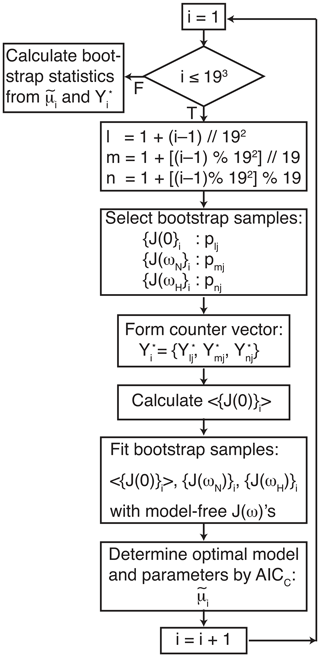Figure 1Flow chart for bootstrap aggregation for the model-free formalism. Indices l, m, and n are determined for each bootstrap sample from the index i using modulo arithmetic, in which $//$ represents floor division, and % is the modulo (remainder) operation. The three indices l, m, and n select pointer and counter vectors from Table 1. The three pointer vectors are used to generate bootstrap samples for J(0), J(ωN), and J(ωH). The three counter vectors are concatenated to form ${\mathbit{Y}}_{i}^{*}$.

Values of a local τm were optimized for each residue in the well-ordered coiled-coil domain of the protein (residues 26–55). Values of τm for residues in the basic region (residues 3–25) and disordered C-terminus (residues 56–58) were fixed at 17.5 ns, the average value obtained for ordered residues. A similar approach was used by Gill and coworkers in the original analysis of the relaxation data . Local values of τm can be used to determine the overall rotational correlation time or diffusion tensor using established methods . Alternatively, the fitting process could be modified to globally optimize the overall rotational correlation time or diffusion tensor while independently optimizing generalized order parameters and correlation times for individual residues . In this scenario, bootstrap aggregation for the internal dynamical parameters would be performed by the same approach as used herein.

4 Results

The results of the conventional analysis using AICC for model selection and Monte Carlo error estimation are shown in Fig. 2. Each of the Monte Carlo simulations was analyzed using the optimal model determined from the original data. The optimal parameters differ slightly from those reported by Gill and coworkers because the present approach used a different spectral density function and model selection method compared to the earlier work . The results of the conventional analysis using AICC for model selection and bootstrap resampling for error estimation are shown in Fig. 3. Each of the bootstrap data sets was analyzed using the optimal model determined from the original data. The results for bootstrap aggregation using AICC to determine the optimal model for each bootstrap sample are shown in Fig. 4. The bootstrap-aggregated smoothed model-free parameters were calculated using Eq. (10), and the smoothed parameter uncertainties were calculated using Eq. (19).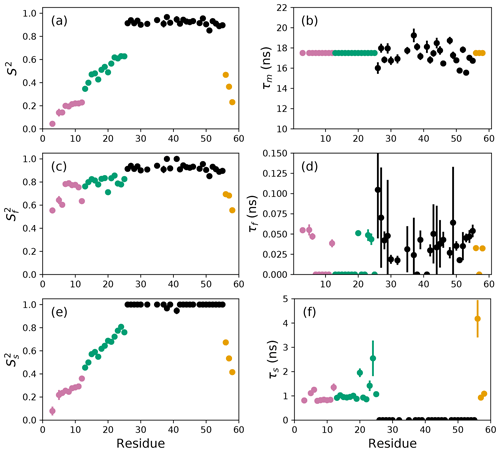Figure 2Model-free parameters from conventional model selection using AICC and 500 Monte Carlo simulations to determine parameter uncertainties. Values of S2, τm, ${S}_{\mathrm{f}}^{\mathrm{2}}$, τf, ${S}_{\mathrm{s}}^{\mathrm{2}}$, and τs are plotted vs. residue number. Overall correlation times (τm) were determined individually for residues in the coiled-coil region (black), while τm was fixed at 17.5 ns for residues in the basic region and C-terminus. Regions of the protein are colored as basic region 1 (residues 3–12) (reddish-purple), basic region 2 (residues 13–25) (green), coiled-coil (residues 26–55) (black), and disordered C-terminus (residues 56–58) (orange) .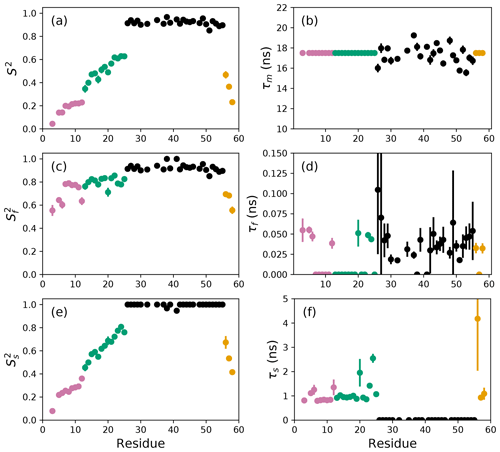Figure 3Model-free parameters from conventional model selection using AICC and bootstrap resampling to determine parameter uncertainties. Values of S2, τm, ${S}_{\mathrm{f}}^{\mathrm{2}}$, τf, ${S}_{\mathrm{s}}^{\mathrm{2}}$, and τs are plotted vs. residue number. Parameter values are identical as in Fig. 2, but the uncertainty estimates differ. Regions of the protein are colored as basic region 1 (residues 3–12) (reddish-purple), basic region 2 (residues 13–25) (green), coiled-coil (residues 26–55) (black), and disordered C-terminus (residues 56–58) (orange) .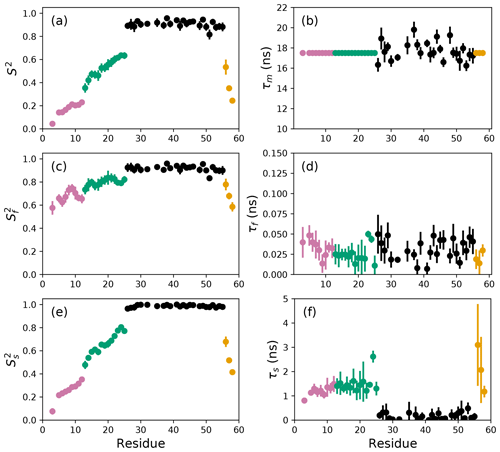Figure 4Smoothed model-free parameters from bootstrap aggregation to determined smoothed parameter estimates and uncertainties. Values of S2, τm, ${S}_{\mathrm{f}}^{\mathrm{2}}$, τf, ${S}_{\mathrm{s}}^{\mathrm{2}}$, and τs are plotted vs. residue number. Regions of the protein are colored as basic region 1 (residues 3–12) (reddish-purple), basic region 2 (residues 13–25) (green), coiled-coil (residues 26–55) (black), and disordered C-terminus (residues 56–58) (orange) .

Bootstrap simulations in which a single optimal model is utilized provide an alternative to Monte Carlo simulations for estimation of (unsmoothed) parameter uncertainties. The uncertainties in $\stackrel{\mathrm{^}}{\mathit{\sigma }}\left({S}^{\mathrm{2}}\right)$ obtained from Monte Carlo simulations and ${\stackrel{\mathrm{^}}{\mathit{\sigma }}}^{*}\left({S}^{\mathrm{2}}\right)$ obtained from conventional bootstrap simulations are compared in Fig. 5a. The uncertainties have approximately the same range but are uncorrelated with each other. These results suggest the non-parametric bootstrap samples simulate the actual data distribution in comparable manner as the parametric Monte Carlo simulations but without assuming a normal distribution of spectral density values. The smoothed parameter uncertainty obtained from Eq. (19) is compared to the uncertainties from Monte Carlo simulations in Fig. 5b. The increase in $\stackrel{\mathrm{̃}}{\mathit{\sigma }}\left({S}^{\mathrm{2}}\right)$ compared to $\stackrel{\mathrm{^}}{\mathit{\sigma }}\left({S}^{\mathrm{2}}\right)$ reflects the effect of model selection uncertainty. As noted by Efron, the estimate of smoothed parameter uncertainty obtained from Eq. (19) is smaller than the naive estimate obtained by applying Eq. (8) to the aggregated bootstrap samples (Efron2014). To illustrate the advantage of Eq. (19), Fig. 5c compares ${\stackrel{\mathrm{^}}{\mathit{\sigma }}}^{u}\left({S}^{\mathrm{2}}\right)$ obtained from Eq. (8) and $\stackrel{\mathrm{̃}}{\mathit{\sigma }}\left({S}^{\mathrm{2}}\right)$ obtained from Eq. (19). Similar trends are observed for other model-free parameters (not shown).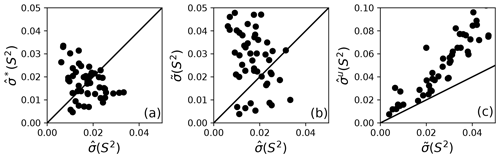Figure 5Comparison of model-free parameter uncertainties. (a) Uncertainties for S2 calculated from Monte Carlo, ${\stackrel{\mathrm{^}}{\mathit{\sigma }}}_{k}$, and bootstrap simulations, ${\stackrel{\mathrm{^}}{\mathit{\sigma }}}_{k}^{*}$, for a single optimal model. (b) Uncertainties for S2 calculated from Monte Carlo simulations for a single optimal model and smoothed ${\stackrel{\mathrm{̃}}{\mathit{\sigma }}}_{k}$ calculated from bootstrap aggregation. (c) Uncertainties ${\stackrel{\mathrm{^}}{\mathit{\sigma }}}_{k}^{u}$ and $\stackrel{\mathrm{̃}}{\mathit{\sigma }}$ for S2 calculated from bootstrap aggregation, illustrating the smaller variability obtained using Eq. (19) for calculation of parameter sample deviations.

Table 2Model selection for selected residues.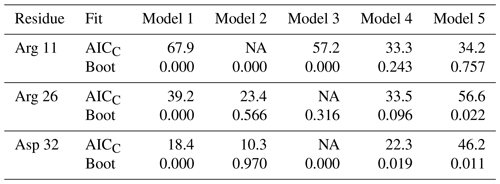For each residue, the top line lists the AICC values determined by fitting the original data to Models 1–5. The second line enumerates the percentage of bootstrap samples for which the indicated model exhibited the lowest AICC. Both Models 2 and 3 contain a single internal effective correlation time. Model 2 is assigned if this correlation time is <0.15 ns (and Model 3 is not assigned, NA). Model 3 is assigned if this correlation time is ≥0.15 ns (and Model 2 is not assigned, NA).

The performance of the conventional analysis, in which a single optimal model is chosen, and bootstrap aggregation, in which parameter values are smoothed over all models, are illustrated for particular residues Arg 11, Arg 26, and Asp 32. Table 2 shows the values of AICC for each model fit to the original spectral density and the percentage that each model was chosen in the bootstrap aggregation. Table 3 shows the optimized model-free parameters for each model fit to the original spectral density data and the smoothed model-free parameters obtained by bootstrap aggregation. The optimal single model selected by AICC is highlighted with an asterisk.

Table 3Model-free parameters for selected residues.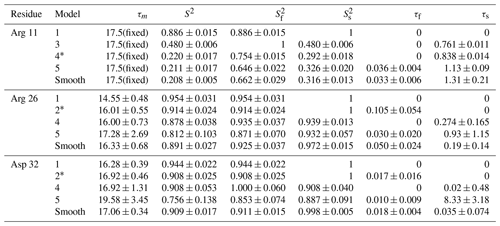For each residue, parameter values for Models 1–5 are calculated from the fit of the original data to the relevant spectral density function, with errors determined by Monte Carlo simulation. The model selected by AICC is indicated by *. Smooth values are obtained by averaging the best fit parameter values across bootstrap samples as in Eq. (10), with errors determined as indicated in Eqs. (15)–(19).

To further illustrate bootstrap aggregation for Arg 11, Arg 26, and Asp 32, Figs. 6, 7, and 8 show the distributions of model-free parameters determined from the optimal model for each bootstrap sample. The calculated spectral density function for bootstrap aggregation is compared to the fitted spectral density functions for each model in Figs. 9, 10, and 11.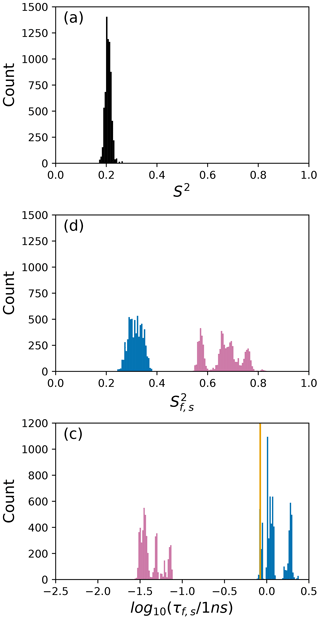Figure 6Distribution of model-free parameters from bootstrap aggregation for residue Arg 11. Color coding is ${S}_{\mathrm{f}}^{\mathrm{2}}$ or τf (reddish-purple) and ${S}_{\mathrm{s}}^{\mathrm{2}}$ or τs (blue). The orange line in (c) indicates the value of τs obtained for the optimal single (unsmoothed) model, Model 4. For clarity, null values of 1 for generalized order parameters and 0 for internal effective correlation times are not shown in the graphs; τf=0 is observed 1664 times.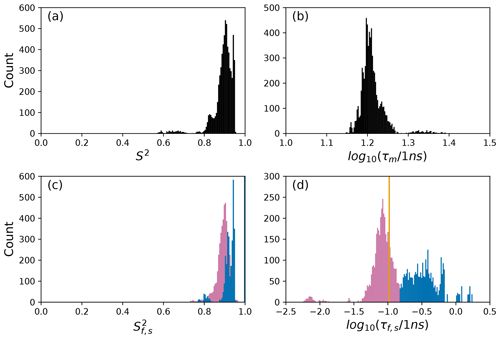Figure 7Distribution of model-free parameters from bootstrap aggregation for residue Arg 26. Color coding is ${S}_{\mathrm{f}}^{\mathrm{2}}$ or τf (reddish-purple) and ${S}_{\mathrm{s}}^{\mathrm{2}}$ or τs (blue). The orange line in (c) indicates the value of τf obtained for the optimal single (unsmoothed) model, Model 2. For clarity, null values of 1 for generalized order parameters and 0 for internal effective correlation times are not shown in the graphs; ${S}_{\mathrm{f}}^{\mathrm{2}}=\mathrm{1}$ is observed 2167 times, ${S}_{\mathrm{s}}^{\mathrm{2}}=\mathrm{1}$ is observed 3884 times, τf=0 is observed 2823 times, and τs=0 is observed 3884 times.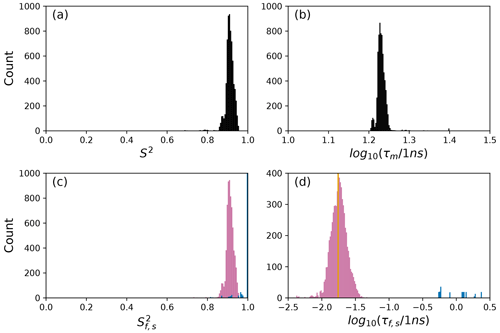Figure 8Distribution of model-free parameters from bootstrap aggregation for residue Asp 32. Color coding is ${S}_{\mathrm{f}}^{\mathrm{2}}$ or τf (reddish-purple) and ${S}_{\mathrm{s}}^{\mathrm{2}}$ or τs (blue). The orange line in (c) indicates the value of τf obtained for the optimal single (unsmoothed) model, Model 2. For clarity, null values of 1 for generalized order parameters and 0 for internal effective correlation times are not shown in the graphs; ${S}_{\mathrm{s}}^{\mathrm{2}}=\mathrm{1}$ was observed 6650 times.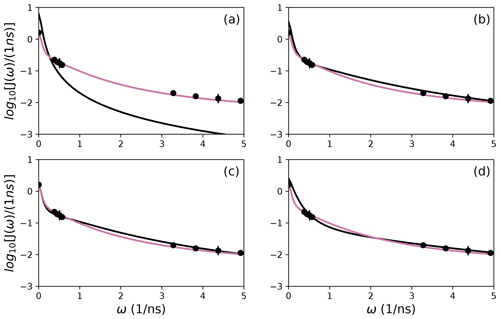Figure 9Comparison of individual fits for Arg 11 of (a) Model 1, (b) Model 3, (c) Model 4, and (d) Model 5 (black lines) or the bootstrap aggregation smoothed fit (reddish-purple line) to (circles) experimental spectral density values.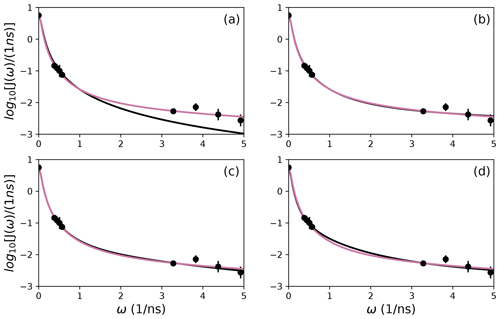Figure 10Comparison of individual fits for Arg 26 of (a) Model 1, (b) Model 3, (c) Model 4, and (d) Model 5 (black lines) or the bootstrap aggregation smoothed fit (reddish-purple line) to (circles) experimental spectral density values.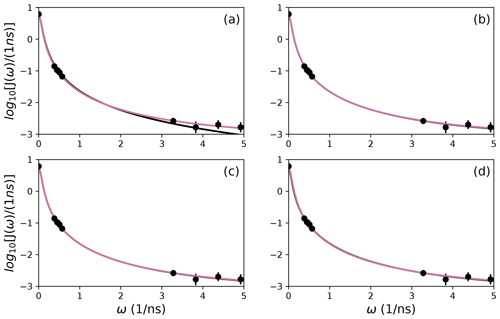Figure 11Comparison of individual fits for Asp 32 of (a) Model 1, (b) Model 3, (c) Model 4, and (d) Model 5 (black lines) or the bootstrap aggregation smoothed fit (reddish-purple line) to (circles) experimental spectral density values.

5 Discussion

The difficulties posed by conventional model selection strategies, in which a single optimal model is chosen using AICC or other fitness statistic, are illustrated for the bZip domain of GCN4 in Fig. 2. In particular, some residues in the basic region (residues 3–25) are analyzed using Model 4, in which τf=0 and other residues are analyzed with Model 5, in which τf>0. The resulting values of the other model-free parameters are systematically affected depending on whether or not τf=0. These systematic effects are evident most clearly in the scatter in ${S}_{\mathrm{f}}^{\mathrm{2}}$ and τs for residues in the basic region. The advantages of bootstrap aggregation in smoothing over variability in model selection is evident in Fig. 4, in which the residue-to-residue variability of the model-free parameters is reduced. Thus, the distributions of τf and τs are much more uniform within the four regions of the protein, suggesting rather uniform timescale processes in each subdomain. The similarity in the distributions for $\stackrel{\mathrm{^}}{\mathit{\sigma }}\left({S}^{\mathrm{2}}\right)$ and ${\stackrel{\mathrm{^}}{\mathit{\sigma }}}^{*}\left({S}^{\mathrm{2}}\right)$, shown in Fig. 5a, indicates that the bootstrap procedure adequately samples the distribution of parameter values. That is, the reduction in parameter variability from bootstrap aggregation does not result from restricted sampling.

The results shown for residue Arg 11 in Tables 2 and 3 and Figs. 6 and 9 illustrate the mechanics behind bootstrap aggregation. The original optimization against the measured data yielded AICC values of 33.3 for Model 4 and 34.1 for Model 5. The conventional analysis then selects Model 4 (with τf=0) as optimal, even though AICC for Model 5 is only slightly larger. In contrast the bootstrap analysis suggests that Model 4 would be optimal for 24 % and Model 5 would be optimal for 76 % of randomly chosen data, under the assumption that the bootstrap samples represent the underlying distribution of spectral density values. Bootstrap smoothing then averages each model-free parameter over the empirical distributions shown in Fig. 6, with resulting optimized spectral density curves compared to the original experimental data in Fig. 9. The results for Model 4 in Table 3 and the corresponding vertical orange line in Fig. 9 show that the selection of Model 4 in the conventional analysis results in an estimate for τs that is skewed toward the lower boundary of the τs bootstrap distribution.

The results shown for residue Arg 26 in Tables 2 and 3 and Figs. 7 and 10 illustrate another advantage of bootstrap aggregation. In this case, the original optimization against the measured data yielded an AICC value of 23.4 for Model 2, substantially smaller than for any other model, implying a single model might be an adequate description for this residue. However, the bootstrap distribution for the internal correlation times is bimodal. The conventional choice of Model 2 results in an estimate of τf roughly centered in the distribution, but the smoothed bootstrap estimates identify the presence of two separable timescales for internal motions, one with a mean of 0.052±0.019 and the other with a mean of 0.13±0.08. Residue 26 is at the juncture between the basic region and coiled-coil motif of the GCN4 bZip domain; consequently, the latter effective internal correlation time might represent a vestige of the more pronounced motions evident in the basic region. The critical value of 0.15 ns used to separate fast from slow motions in the present work was chosen empirically to distinguish the two distributions observed for residue 26 (and used for all other residues). More sophisticated clustering algorithms could be used to make this distinction between Models 2 and 3.

The results shown for residue Asp 32 in Tables 2 and 3 and Figs. 8 and 11 illustrate a case of strong agreement between the conventional analysis and bootstrap aggregation when a single motional model is strongly favored by the experimental data. The distributions shown in Fig. 8 then represent the variability in model-free parameters across the bootstrap samples. These results would be comparable to results obtained in Fig. 3, in which the bootstrap samples were used to estimate model-free parameter uncertainties ${\stackrel{\mathrm{^}}{\mathit{\sigma }}}_{k}^{*}$ for a single fixed optimal model.

The present application of bootstrap aggregation used spin relaxation data recorded at four static magnetic fields. A total of 6859 bootstrap samples were used to calculate smoothed parameter estimates. Data recorded at three static magnetic fields provide nine spectral density values but allow only 73=343 bootstrap samples. To test the effect of such a drastic reduction in the size of the bootstrap sample, the relaxation rate constants recorded at 600, 800, and 900 MHz were analyzed for the disordered basic region (residues 3–25). This preserves the same range of sampled frequencies as for the original analysis, but only seven spectral density values are obtained for each residue, after averaging the three values of J(0). The smaller number of spectral density values results in smaller numbers of degrees of freedom when fitting the model-free spectral density models. As a consequence, only Models 3 and 4 were selected for the basic region in the conventional analysis; essentially, the data were not sufficient to determine τf and τs simultaneously (Model 5). Nonetheless, bootstrap aggregation was effective in smoothing the effects of model selection error between Models 3 and 4, even with only 343 bootstrap samples (not shown). A number of studies have investigated the number of model parameters that can be determined from backbone amide 15N relaxation data recorded at high static magnetic fields . The present results suggest that measurements at four static magnetic fields are required to fully statistically characterize the information content of such measurements within the extended model-free formalism.

6 Conclusions

Model selection error is a classical problem in statistics and has been recognized as a concern in the model-free analysis of NMR spin relaxation data since the work of . Bootstrap aggregation has emerged as a powerful approach for incorporating selection error into statistical model-building . However, bootstrap aggregation requires sufficient numbers of data points to allow faithful resampling of the distribution of the data. This issue is made more serious by the nature of nuclear spin relaxation data: spectral density values for J(0), J(ωN), and J(0.87ωH) are very different and should not be interchanged by resampling. As shown in the present work, resampling within blocks of spectral density values clustered as J(0), J(ωN), and J(0.87ωH) recorded at three or four static magnetic fields is sufficient to enable bootstrap aggregation. However, the larger data set available from four static magnetic fields allows more reliable resolution of two internal correlation times, τf<0.15 ns and τs≥0.15 ns.

Aggregation improves parameter stability by averaging over all models represented in the bootstrap sample. As applied to 15N spin relaxation data for the bZip domain of GCN4, bootstrap aggregation reduces residue-to-residue variations in optimal model-free parameters, particularly in the partially disordered basic region. Consequently, trends in the conformational dynamics along the polypeptide backbone that reflect actual physical properties of the protein become more evident. Notably, local maxima in generalized order parameters within the basic region (residues 3–25), most evident for residues 8 and 9 and for residues 14 and 15 in Fig. 4, reflect transient populations of helical conformations observed in molecular dynamics simulations . NMR spin relaxation spectroscopy is a powerful approach for interrogating conformational dynamics of biological macromolecules. Bootstrap aggregation, coupled with experimental NMR spin relaxation measurements at multiple static magnetic fields, promises to advance efforts to understand the interplay between conformation and function in biology.

Code and data availability

A Jupyter notebook (Python 3.6) is provided for performing all data analyses reported in the publication. The NMR data analyzed in the publication are available at Mendeley Data (https://doi.org/10.17632/vpwz6mrynr.1; ).

Supplement

Author contributions

AGP conceived the project. Calculations and writing of the paper were performed by TC and AGP.

Competing interests

The authors declare that they have no conflict of interest.

Special issue statement

Acknowledgements

Arthur G. Palmer III acknowledges the support from the National Institutes of Health. Some of the work presented here was conducted at the Center on Macromolecular Dynamics by NMR Spectroscopy located at the New York Structural Biology Center, supported by the NIH National Institute of General Medical Sciences. Arthur G. Palmer III is a member of the New York Structural Biology Center. This paper is dedicated to Prof. Geoffrey Bodenhausen on the occasion of his 70th birthday.

Financial support

This research has been supported by the National Institutes of Health (grant nos. R35GM130398 and P41GM118302).

Review statement

This paper was edited by Malcolm Levitt and reviewed by three anonymous referees.

References

Abergel, D., Volpato, A., Coutant, E. P., and Polimeno, A.: On the reliability of NMR relaxation data analyses: A Markov Chain Monte Carlo approach, J. Magn. Reson., 246, 94–103, 2014. a

Abyzov, A., Salvi, N., Schneider, R., Maurin, D., Ruigrok, R. W., Jensen, M. R., and Blackledge, M.: Identification of dynamic modes in an intrinsically disordered protein using temperature-dependent NMR relaxation, J. Am. Chem. Soc., 138, 6240–6251, 2016. a

Box, G. E. P.: Science and statistics, J. Am. Stat. Assoc., 71, 791–799, 1976. a

Breiman, L.: Bagging predictors, Mach. Learn., 24, 123–140, 1996. a

Buja, A. and Stuetzle, W.: Observations on bagging, Stat. Sin., 16, 323–351, 2006. a, b

Calandrini, V., Abergel, D., and Kneller, G. R.: Fractional protein dynamics seen by nuclear magnetic resonance spectroscopy: Relating molecular dynamics simulation and experiment, J. Chem. Phys., 133, 145101, https://doi.org/10.1063/1.3486195, 2010. a

Chen, J., Brooks C.L., 3rd, and Wright, P. E.: Model-free analysis of protein dynamics: assessment of accuracy and model selection protocols based on molecular dynamics simulation, J. Biomol. NMR, 29, 243–257, 2004. a

Clore, G. M., Szabo, A., Bax, A., Kay, L. E., Driscoll, P. C., and Gronenborn, A. M.: Deviations from the simple two-parameter model-free approach to the interpretation of nitrogen-15 nuclear magnetic relaxation of proteins, J. Am. Chem. Soc., 112, 4989–4991, 1990. a

d'Auvergne, E. J. and Gooley, P. R.: The use of model selection in the model-free analysis of protein dynamics, J. Biomol. NMR, 25, 25–39, 2003. a

d'Auvergne, E. J. and Gooley, P. R.: Set theory formulation of the model-free problem and the diffusion seeded model-free paradigm, Mol. Biosyst., 3, 483–494, 2007. a, b

d'Auvergne, E. J. and Gooley, P. R.: Optimisation of NMR dynamic models I. Minimisation algorithms and their performance within the model-free and Brownian rotational diffusion spaces, J. Biomol. NMR, 40, 107–119, 2008a. a, b

d'Auvergne, E. J. and Gooley, P. R.: Optimisation of NMR dynamic models II. A new methodology for the dual optimisation of the model-free parameters and the Brownian rotational diffusion tensor, J. Biomol. NMR, 40, 121–133, 2008b. a, b

Efron, B.: Estimation and accuracy after model selection, J. Am. Stat. Assoc., 109, 991–1007, 2014. a, b, c, d, e, f

Farrow, N., Zhang, O., Szabo, A., Torchia, D., and Kay, L.: Spectral density function mapping using 15N relaxation data exclusively, J. Biomol. NMR, 6, 153–162, 1995. a, b

Gill, M. L., Byrd, R. A., and Palmer, A. G.: Dynamics of GCN4 facilitate DNA interaction: a model-free analysis of an intrinsically disordered region, Phys. Chem. Chem. Phys., 18, 5839–5849, 2016. a, b, c, d, e, f, g, h, i, j, k, l, m

Gill, M. L., Byrd, R. A., and Palmer, A. G.: Data for: Dynamics of GCN4 facilitate DNA interaction: a model-free analysis of an intrinsically disordered region, Mendeley Data [data set], V1, https://doi.org/10.17632/vpwz6mrynr.1, 2021. a

Halle, B. and Wennerström, H.: Interpretation of magnetic resonance data from water nuclei in heterogeneous systems, J. Chem. Phys., 75, 1928–1943, 1981. a

Hsu, A., Ferrage, F., and Palmer, A. G.: Analysis of NMR spin-relaxation data using an inverse Gaussian distribution function, Biophys. J., 115, 2301–2309, 2018. a

Hsu, A., Ferrage, F., and Palmer, A. G.: Correction: Analysis of NMR spin-relaxation data using an inverse Gaussian distribution function, Biophys. J., 119, 884–885, 2020. a

Hurvich, C. M. and Tsai, C.-L.: Regression and time series model selection in small samples, Biometrika, 76, 297–307, 1989. a

Khan, S. N., Charlier, C., Augustyniak, R., Salvi, N., Déjean, V., Bodenhausen, G., Lequin, O., Pelupessy, P., and Ferrage, F.: Distribution of pico- and nanosecond motions in disordered proteins from nuclear spin relaxation, Biophys. J., 109, 988–999, 2015. a, b

Kroenke, C., Loria, J. P., Lee, L., Rance, M., and Palmer, A. G.: Longitudinal and transverse 1H-15N dipolar/15N chemical shift anisotropy relaxation interference: unambiguous determination of rotational diffusion tensors and chemical exchange effects in biological macromolecules, J. Am. Chem. Soc., 120, 7905–7915, 1998. a

Lee, L., Rance, M., Chazin, W., and Palmer, A.: Rotational diffusion anisotropy of proteins from simultaneous analysis of 15N and 13Cα nuclear spin relaxation, J. Biomol. NMR, 9, 287–298, 1997. a, b

Lemaster, D. M.: Larmor frequency selective model free analysis of protein NMR relaxation, J. Biomol. NMR, 6, 366–374, 1995. a

Lipari, G. and Szabo, A.: Model-free approach to the interpretation of nuclear magnetic resonance relaxation in macromolecules. 1. Theory and range of validity, J. Am. Chem. Soc., 104, 4546–4559, 1982a. a

Lipari, G. and Szabo, A.: Model-free approach to the interpretation of nuclear magnetic resonance relaxation in macromolecules. 2. Analysis of experimental results, J. Am. Chem. Soc., 104, 4559–4570, 1982b. a

Mandel, A. M., Akke, M., and Palmer, A. G.: Backbone dynamics of Escherichia coli ribonuclease HI: Correlations with structure and function in an active enzyme, J. Mol. Biol., 246, 144–163, 1995. a, b, c

Mendelman, N. and Meirovitch, E.: SRLS analysis of 15N–1H NMR relaxation from the protein S100A1: Dynamic structure, calcium binding, and related changes in conformational entropy, J. Phys. Chem. B, 125, 805–816, 2021. a

Mendelman, N., Zerbetto, M., Buck, M., and Meirovitch, E.: Conformational entropy from mobile bond vectors in proteins: A viewpoint that unifies NMR relaxation theory and molecular dynamics simulation approaches, J. Phys. Chem. B, 124, 9323–9334, 2020. a

Ollila, O. H. S., Heikkinen, H. A., and Iwaï, H.: Rotational dynamics of proteins from spin relaxation times and molecular dynamics simulations, J. Phys. Chem. B, 122, 6559–6569, 2018. a

Palmer, A. G., Rance, M., and Wright, P. E.: Intramolecular motions of a zinc finger DNA-binding domain from xfin characterized by proton-detected natural abundance 13C heteronuclear NMR spectroscopy, J. Am. Chem. Soc., 113, 4371–4380, 1991. a

Polimeno, A., Zerbetto, M., and Abergel, D.: Stochastic modeling of macromolecules in solution. I. Relaxation processes, J. Chem. Phys., 150, 184107, https://doi.org/10.1063/1.5077065, 2019a. a

Polimeno, A., Zerbetto, M., and Abergel, D.: Stochastic modeling of macromolecules in solution. II. Spectral densities, J. Chem. Phys., 150, 184108, https://doi.org/10.1063/1.5077066, 2019b. a

Robustelli, P., Trbovic, N., Friesner, R. A., and Palmer, A. G.: Conformational dynamics of the partially disordered yeast transcription factor GCN4, J. Chem. Theor. Comput., 9, 5190–5200, 2013. a

Smith, A. A., Ernst, M., Meier, B. H., and Ferrage, F.: Reducing bias in the analysis of solution-state NMR data with dynamics detectors, J. Chem. Phys., 151, 034102, https://doi.org/10.1063/1.5111081, 2019.  a

Stone, M. J., Fairbrother, W. J., Palmer, A. G., Reizer, J., Saier, M. H., and Wright, P. E.: The backbone dynamics of the Bacillus subtilis glucose permease IIA domain determined from 15N NMR relaxation measurements, Biochemistry, 31, 4394–4406, 1992. a

Tugarinov, V., Liang, Z. C., Shapiro, Y. E., Freed, J. H., and Meirovitch, E.: A structural mode-coupling approach to 15N NMR relaxation in proteins, J. Am. Chem. Soc., 123, 3055–3063, 2001. a

Zerbetto, M., Anderson, R., Bouguet-Bonnet, S., Rech, M., Zhang, L., Meirovitch, E., Polimeno, A., and Buck, M.: Analysis of 15N–1H NMR relaxation in proteins by a combined experimental and molecular dynamics simulation approach: Picosecond-nanosecond dynamics of the Rho GTPase binding domain of plexin-B1 in the dimeric state indicates allosteric pathways, J. Phys. Chem. B, 117, 174–184, 2013. a